# Conductor

Ideal linear electrical conductor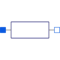# Information

This information is part of the Modelica Standard Library maintained by the Modelica Association.

The linear conductor connects the branch voltage v with the branch current i by i = v*G. The Conductance G is allowed to be positive, zero, or negative.

# Parameters (5)

useHeatPort Value: false Type: Boolean Description: =true, if heatPort is enabled Value: T_ref Type: Temperature (K) Description: Fixed device temperature if useHeatPort = false Value: Type: Conductance (S) Description: Conductance at temperature T_ref Value: 300.15 Type: Temperature (K) Description: Reference temperature Value: 0 Type: LinearTemperatureCoefficient (¹/K) Description: Temperature coefficient of conductance (G_actual = G_ref/(1 + alpha*(T_heatPort - T_ref))

# Connectors (3)

p n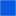Type: PositivePin Description: Positive electrical pin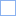Type: NegativePin Description: Negative electrical pin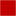Type: HeatPort_a Description: Conditional heat port

# Used in Examples (2)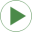ChuaCircuit Modelica.Electrical.Analog.Examples Chua's circuit, ns, V, AResonanceCircuits Modelica.Electrical.Analog.Examples Resonance circuits: example to demonstrate generation of FMUs (Functional Mock-up Units)

# Used in Components (4)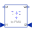Conductor Modelica.Electrical.Analog.Examples.Utilities Input/output block of a conductance model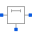OLine Modelica.Electrical.Analog.Lines Lossy Transmission Line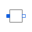segment Modelica.Electrical.Analog.Lines.M_OLine Multiple line segment model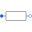Conductor Modelica.Electrical.MultiPhase.Basic Ideal linear electrical conductors